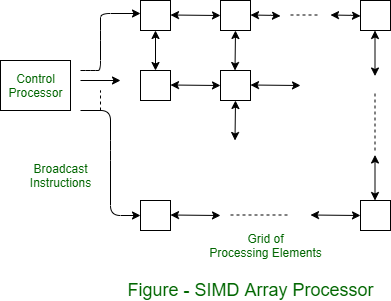Open in App
Not now

# Operation of SIMD Array Processor

• Difficulty Level : Medium
• Last Updated : 10 Nov, 2022

The SIMD form of parallel processing is called Array processing. Figure shows the array processor. A two-dimensional grid of processing elements transmits an instruction stream from a central control processor. As each instruction is transmitted, all elements execute it simultaneously. Each processing element is connected to its four nearest neighbors for the purposes of data exchange. Connections around the end can be provided on both rows and columns, but they are not shown in figure.It is instructive to consider a specific calculation to understand the capabilities of the SIMD architecture. Grids of processing elements can be used to solve two-dimensional problems. For example, if each element of the grid represents a point in space, the array can be used to calculate the temperature at points in the interior of a conduction plane.

Suppose the edges of the plane are held at certain temperatures. An approximate solution at discrete points represented by processing elements is as follows. The outer edges are initialized at a specified temperature. All internal points are initial to some arbitrary values, not necessarily equal. Iterations are then executed in parallel in each element. Each iteration involves calculating a better estimate of temperature at a point by averaging the current values of its four nearest neighbors. Processes stop when ordinal estimates are closer than some predetermined small difference.

The capacity required in array processors to perform such calculations is quite simple. Each element must be able to exchange values with each of its neighbors on the paths shown in figure. Each processing element has some registers and some local memory to store the data. It also has a register, called a network register, which is used to facilitate the movement of values from its neighbors. The central processor can broadcast an instruction to move values across the network, registering a step up, down, left, or right.

Each processing element also has an ALU to execute arithmetic instructions transmitted by the control processor. Using these features, a sequence of instructions can be transmitted repeatedly to implement iterative loops. The control processor must be able to determine that each element of the processing has developed its own component of temperature to the required accuracy. To do this, each element sets the internal status bit to 1 to indicate this condition. The grid interconnect includes a feature that allows the controller to detect that all status bits have been set at the end of an iteration.

Array processors are highly specialized machines. They are well-suited numerical problems that can be expressed in matrix or vector format. How they are not very useful in speeding up general computations.

My Personal Notes arrow_drop_up